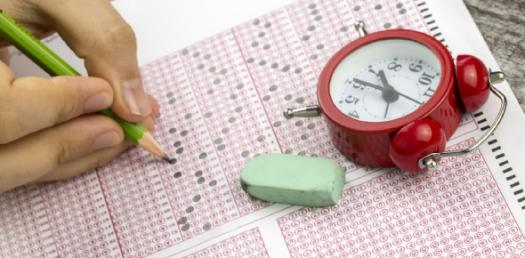# The Official Phy103 Practice Test

40 Questions | Attempts: 808
ShareSettingsThis test was set up by Oyewole Kolawole David Of Mechatronics Engineering department, Fuoye, to help the 100L students prepare for their Physics Exam

• 1.
The pressure at any given point of a non-moving fluid is called the ____________
• A.

Guage Pressure

• B.

Atmospheric Pressure

• C.

Differential Pressure

• D.

Hydrostatic Pressure

• 2.
_________ is the evidence that matter is made up of atoms and particles that are in constant vibration
• A.

Brownian motion

• B.

Accelerated motion

• C.

Bernuolli principle

• D.

Special relativity

• 3.
Mercury melts at ________
• A.

0° - 4°C

• B.

404°C

• C.

-38.829°C

• D.

112°C

• 4.
Bulk Modulus, Pressure, Force, Stress – Which one of these won’t have the same unit as the others?
• A.

Bulk Modulus

• B.

Pressure

• C.

Force

• D.

Stress

• 5.
Which of the following is true?
• A.

Flow is laminar inside the boundary layer and turbulent outside

• B.

Flow is turbulent inside the boundary layer and laminar outside

• C.

Flow is laminar both inside and outside of the boundary layer

• D.

Flow is turbulent both inside and outside of the boundary layer

• 6.
The SI unit of Surface tension is ______
• A.

Nm

• B.

N/m

• C.

Kg/cm

• D.

Pa

• 7.
__________ is a pointer for the direction of energy transfer.
• A.

Thermodynamics

• B.

Weight

• C.

Temperature

• D.

Thermocouple

• 8.
Which thermometer adopts pressure as its thermometric property?
• A.

Alcohol in gas Thermometer

• B.

Electric Resistance

• C.

Thermocouple

• D.

Constant volume gas thermometer

• 9.
Which of the following is not a scale used in measuring temperature?
• A.

• B.

Fahrenheit

• C.

Temperature

• D.

None of the above

• 10.
The scale of 212°F in °C is ___
• A.

89°C

• B.

100°C

• C.

88°C

• D.

68.90°C

• 11.
Water strider can walk walk on water because
• A.

It's weight is not enough to penetrate the surface

• B.

The Viscosity of the water is High

• C.

The surface tension of water strider is High

• D.

It is not true,

• 12.
In the Zeroth law of thermodynamics, the third body stand for the
• A.

Thermocouple

• B.

Thermodynamics

• C.

Thermostatic body

• D.

Thermometer

• 13.
_______ is the part of the universe, we choose to study
• A.

Isolated System

• B.

Thermodynamic cycle

• C.

System

• D.

Surrounding

• 14.
For an isolated system
• A.

Neither the mass nor energy can transfer between the system and the surrounding

• B.

The Thermometer is always stagnant

• C.

There is no acceleration

• D.

Entropy

• 15.
Which of the laws of thermodynamics defines entropy
• A.

Zeroth

• B.

First

• C.

Second

• D.

Third

• 16.
First law of thermodynamics deals with
• A.

Conservation of mass

• B.

Conservation of momentum

• C.

Conservation of energy

• D.

Conservation of pressure

• 17.
The equation for the average kinetic energy is_________
• A.

0.5KT

• B.

1.5KT

• C.

3.5KT

• D.

3.2KT

• 18.
What is the dimension of strain
• A.

L x L

• B.

It's dimensionless

• C.

M X L X T

• D.

M/L

• 19.
An object has a mass of 10kg and a length of 4m, given that the cross-sectional area of the object is 32.5m^2, Now calculate the density of the object
• A.

0.77kg/m^3

• B.

0.077kg/m^3

• C.

7.077kg/m^3

• D.

0.0077kg/m^3

• 20.
A body weighs 0.3N in air, 0.25N when fully immersed in water and 0.27N when fully immersed in a liquid. Calculate its loss of weight in water
• A.

0.05N

• B.

0.003N

• C.

0.6N

• D.

1.3N

• 21.
A hydraulic press contains 0.25m^3 of oil find the decrease in the volume of the oil when it's subjected to a pressure increase (P' - P) = 1.6 x  10 ^7 Pa. The bulk Modulus of the oil is 5.0GigaPa
• A.

0.6796

• B.

0.0008

• C.

0.00008

• D.

0.008

• 22.
A uniform cylindrical wooden block floats in water with 1/3 of its height above the water level. In a liquid of a relative density 0.8, what fraction of its height will be above the liquid
• A.

1/6v

• B.

1/7v

• C.

1/4v

• D.

1/3v

• 23.
When a leaf floats on the surface of water, its able to float because of the force on the surface of the water, and this force is called
• A.

Young modulus

• B.

Density

• C.

Viscosity

• D.

Surface Tension

• 24.
Which among the following is an assumption of Hagen-Poiseuille equation?
• A.

Fluid is compressible

• B.

Fluid is uniform

• C.

Fluid is laminar

• D.

Fluid is turbulent

• 25.
Which of the following affects surface tension
• A.

Thermodynamics

• B.

Type of force

• C.

Impurity

• D.

Pressure

## Related TopicsBack to top
×

Wait!
Here's an interesting quiz for you.